Browse by Topic
Related Topics

# Quantum Chemistry: Step-by-Step Chemistry Series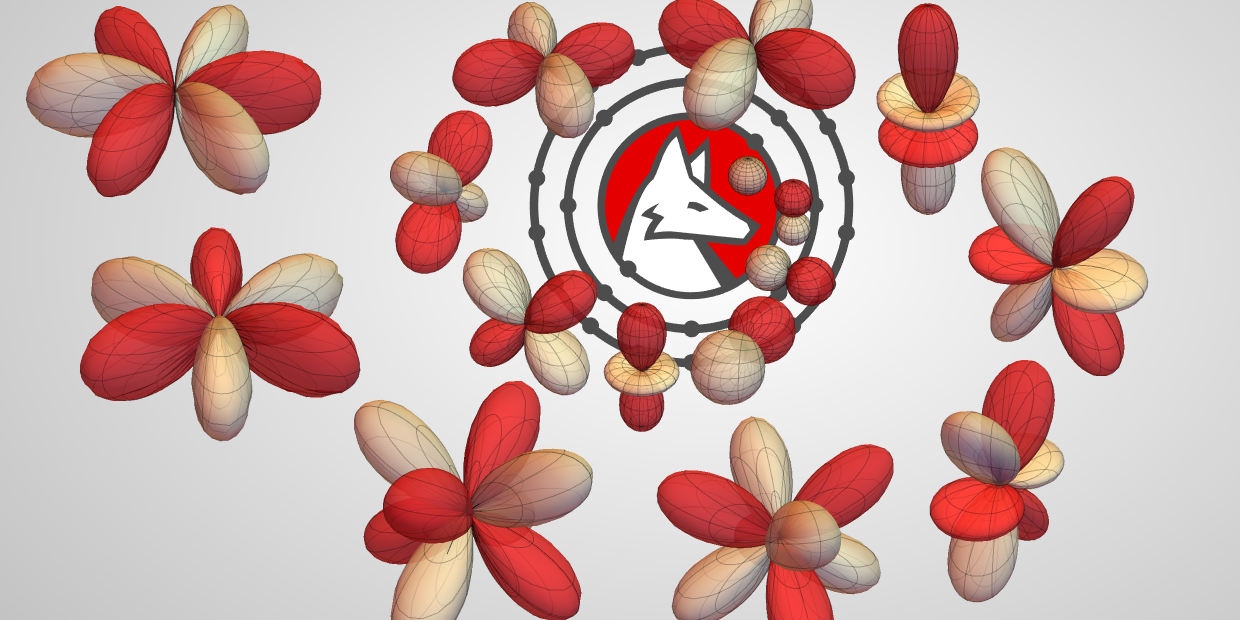After working our way through chemical reactions, solutions and structure and bonding, we close out our step-by-step chemistry series with quantum chemistry. Quantum chemistry is the application of quantum mechanics to atoms and molecules in order to understand their properties.

Have you ever wondered why the periodic table is structured the way it is or why chemical bonds form in the first place? The answers to those questions and many more come from quantum chemistry. Wolfram|Alpha and its step-by-step chemistry offerings won’t make the wave-particle duality any less weird, but they will help you connect chemical properties to the underlying quantum mechanical behavior.

The step-by-step solutions provide stepwise guides that can be viewed one step at a time or all at once while working through a problem. Read on for example problems covering orbital diagrams, frequency and wavelength conversions, and mass-energy equivalence.

## Orbital Diagrams

One fundamental aspect of chemistry is understanding where electrons live in atoms. Building orbital diagrams provides a good way to visualize this information. The step-by-step solution provides a general framework for solving this class of problems in the Plan step. Details of how to represent the information graphically, along with explanations of core electrons, are provided. An explanation of how many electrons a given orbital set can hold is available via the “Show intermediate steps” button.

### Example Problem

Build the orbital diagram for elemental iron.

### Step-by-Step Solution

For this class of problem, just enter “orbital diagram for elemental iron”.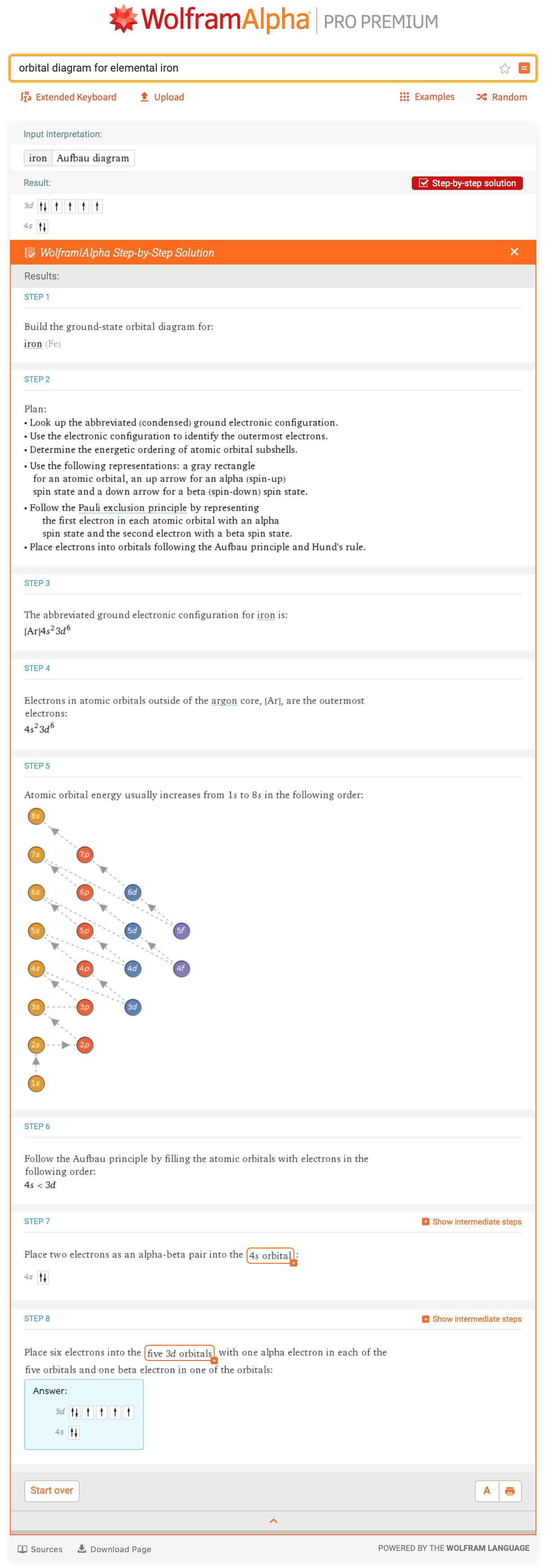## Frequency & Wavelength Conversion

Electromagnetic radiation is central to many techniques in analytical chemistry. Converting frequency and wavelength is a critical skill for understanding theoretical models and interpreting experimental spectra. The photon wavelength calculator provides instructions for interconversion of the frequency and wavelength of electromagnetic radiation.

### Example Problem

A sodium streetlight gives off yellow light with a wavelength of 598 nm. What is the frequency of this light?

### Step-by-Step Solution

The calculator can be fed known information directly via “photon wavelength lambda=598 nm”.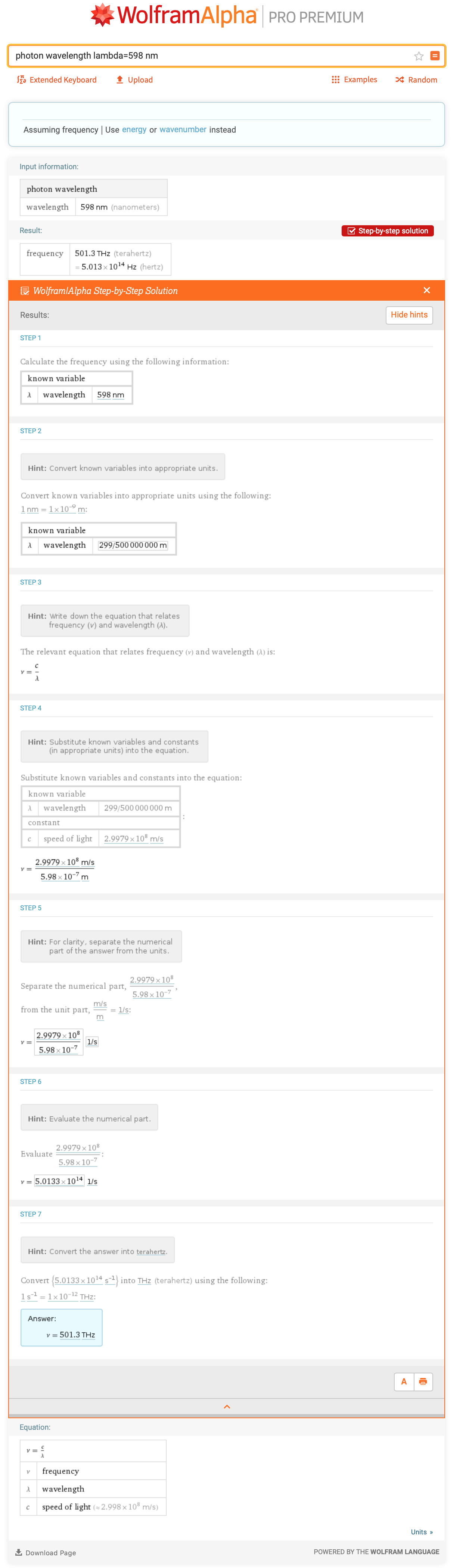## Mass-Energy Equivalence

The nuclear binding energy is useful when tracking energy changes in nuclear reactions. Converting between mass and energy is a key step in computing nuclear binding energies. The relativistic energy calculator provides instructions for converting between mass and energy.

### Example Problem

The mass defect for a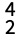He nucleus is 0.0304 u. What is the binding energy for this nuclide in joules per nucleus and MeV per nucleus?

### Step-by-Step Solution

The calculator can be fed known information directly via “relativistic energy m=0.0304 u”.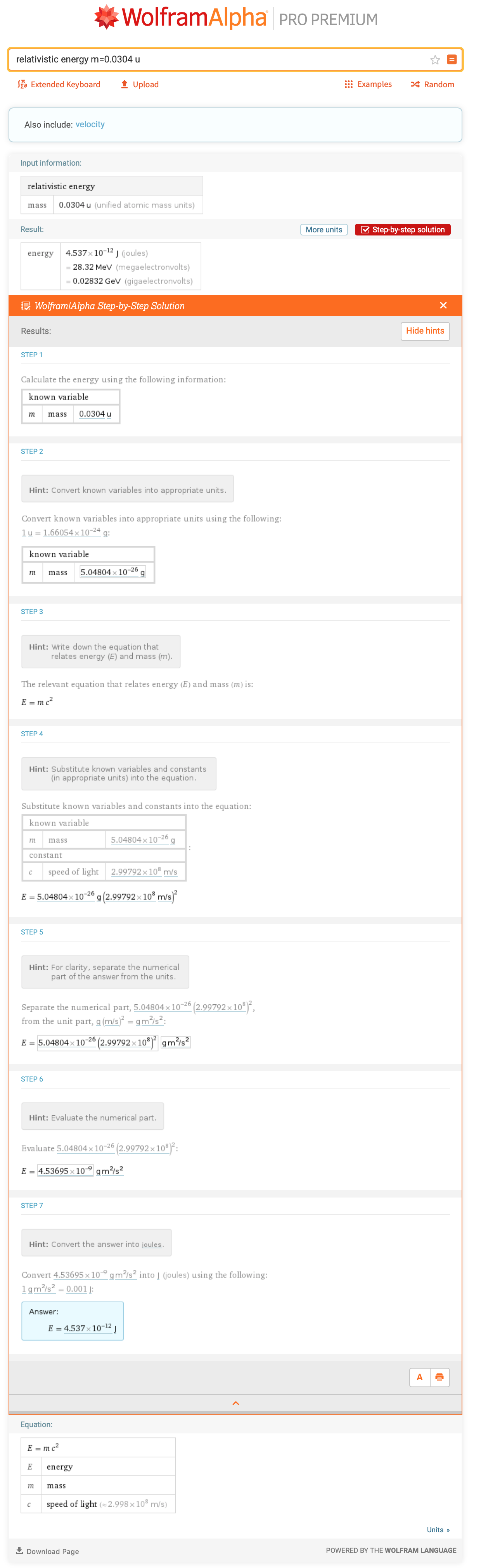## Challenge Problems

Test your problem-solving skills by using the Wolfram|Alpha tools described to solve these word problems on quantum chemistry. Answers will be provided at the end of this post!

1. Use an orbital diagram to predict the electron configuration of the P3– anion.
2. The Trinity test released 5.5 × 1026 MeV. What mass is equivalent to this energy?

## Answers to Last Week’s Challenge Problems

Here are the answers to last week’s challenge problems on structure and bonding.

### 1. What is the oxidation state of hydrogen in lithium aluminum hydride?

Recall that oxidation state and oxidation number are the same. Additionally, recall that Wolfram|Alpha computes all oxidation numbers in a molecule. Note that “hydrogen oxidation state lithium aluminum hydride” actually returns results for both hydrogen (H2) and lithium aluminum hydride.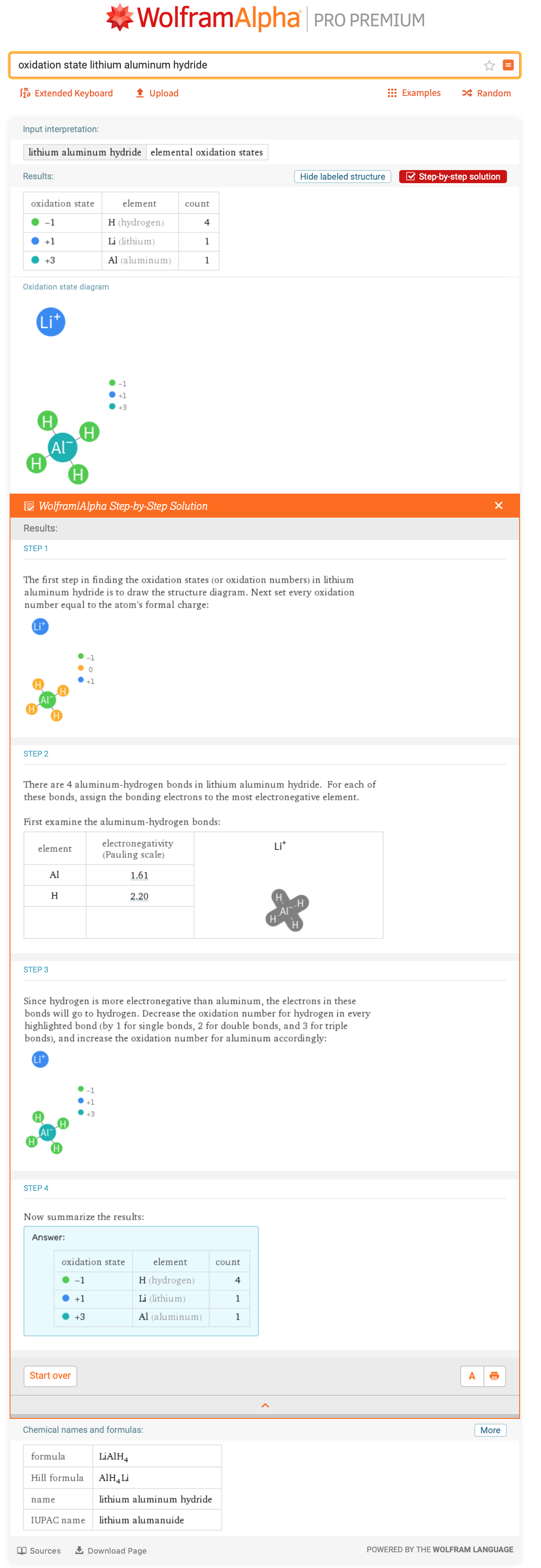### What is the orbital hybridization of the central atom in SF6?

Wolfram|Alpha determines the hybridization for all elements except hydrogen (it only has one orbital and therefore cannot hybridize) in a molecule. So you would just need to determine that S is the central atom.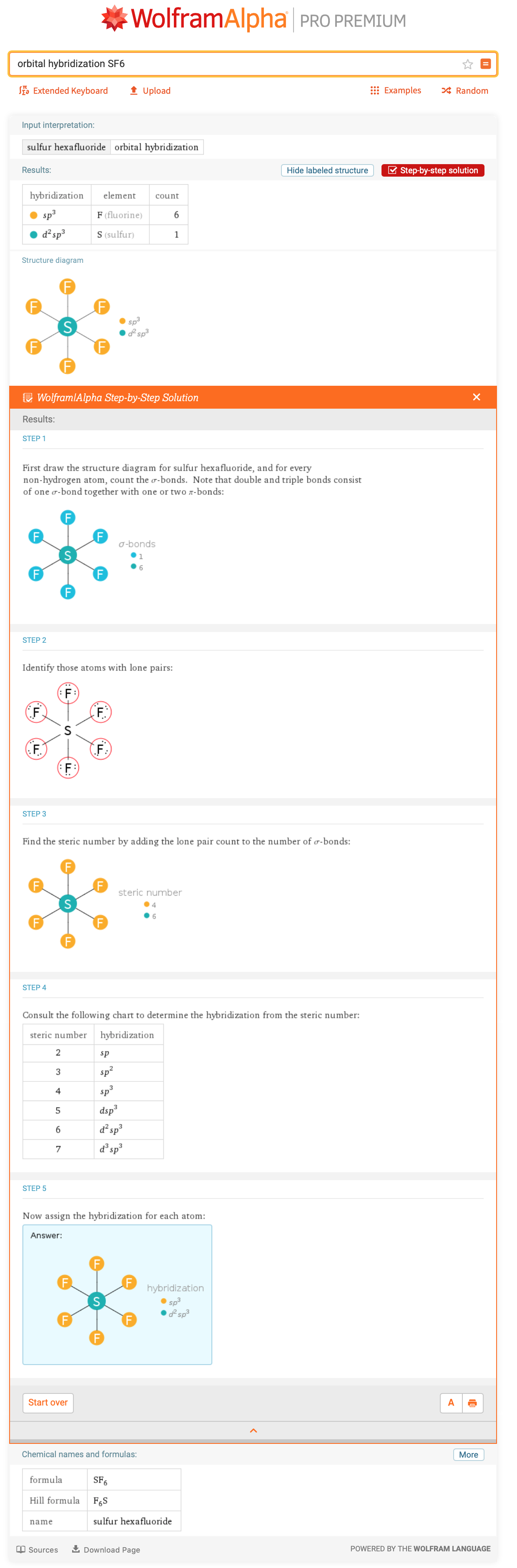## Answers to the Quantum Chemistry Challenge Problems

### 1. Use an orbital diagram to predict the electron configuration of the P3– anion.

Wolfram|Alpha generates orbital diagrams for neutral atoms in their ground state. However, the neutral atom diagram can be used to figure out where additional electrons will go or which electrons might be removed the easiest. In this case, three extra electrons need to be added to make the trianion.

The electron configuration for the phosphorus trianion is 3s2 3p6.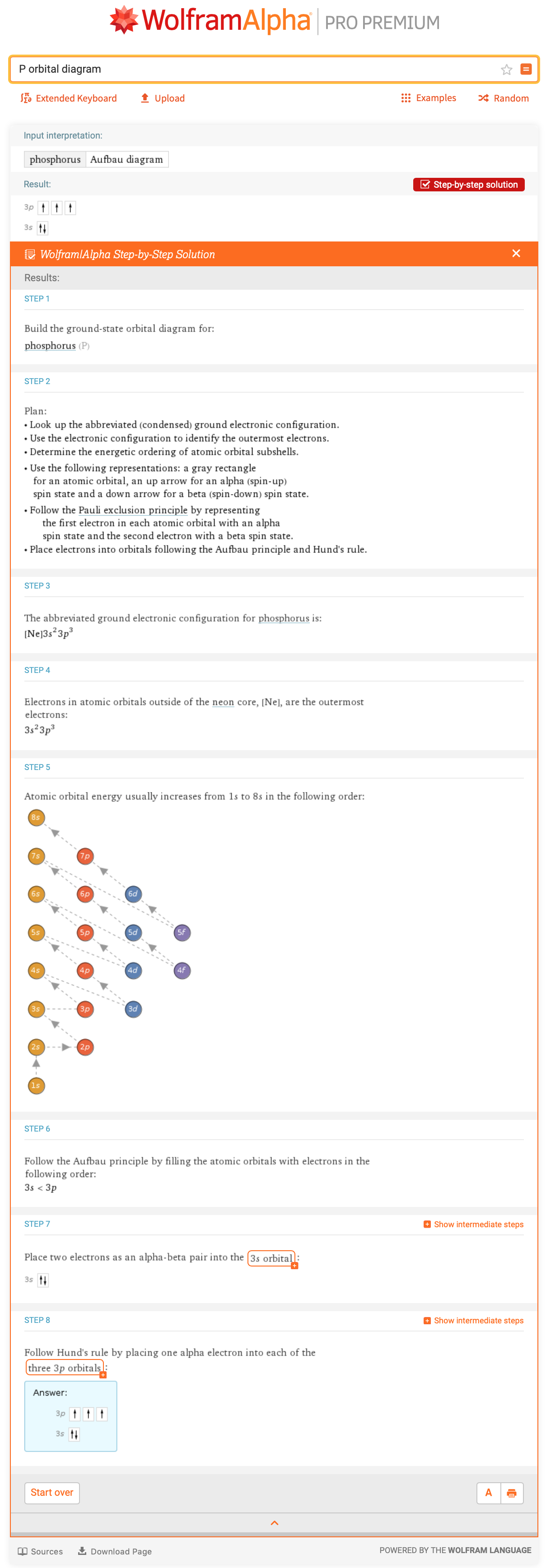### 2. The Trinity test released 5.5 x 1026 MeV. What mass is equivalent to this energy?

The mass-energy equivalence calculator can be used to solve this, but now the energy must be passed in rather than the mass.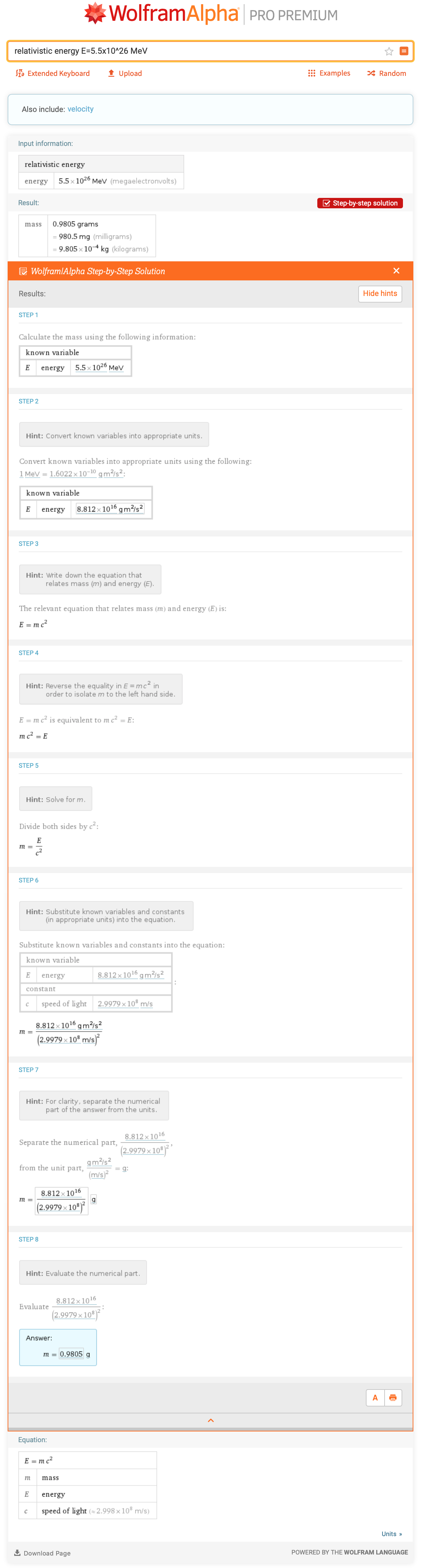We hope you’ve enjoyed reading our step-by-step chemistry series, and that our review of chemical reactions, solutions and structure and bonding, along with today’s post on quantum chemistry, have been useful in your studies. New step-by-step solution offerings for chemistry are always rolling out; equilibrium constant expressions, rate-of-reaction expressions, electron configurations, valence electrons, reaction thermochemistry and solution pH are just some of the areas on the to-do list. So stay tuned and check back frequently!